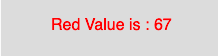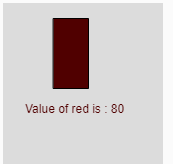# p5.js red() function

The red() function in p5.js is used to extract the red value from a color or pixel array.

Syntax:

`red(c)`

Parameters: This function accepts single parameter c which stores the p5.Color object, color components, or CSS color.

Below programs illustrates the red() function in p5.js:

Example 1: This example uses red() function to extract the red value from the pixel array.

 `function` `setup() { ` ` `  `    ``// Create Canvas of size 300*80 ` `    ``createCanvas(300, 80); ` `} ` ` `  `function` `draw() { ` `     `  `    ``// Set background color ` `    ``background(220); ` `     `  `    ``// Initialize the parameter ` `    ``let c = color(67, 126, 255, 102); ` `     `  `    ``// Extract the red value ` `    ``let y = red(c); ` `     `  `    ``// Set the font size ` `    ``textSize(16); ` ` `  `    ``// Set the font color ` `    ``fill(color(``'red'``)); ` `     `  `    ``// Display result ` `    ``text(``"Red Value is : "` `+ y, 50, 30); ` `} `

Output:Example 2: This example uses red() function to extract the red value from the pixel array and used as fill color.

 `function` `setup() { ` ` `  `    ``// Create Canvas of size 300*180 ` `    ``createCanvas(160, 180); ` `} ` ` `  `function` `draw() { ` `     `  `    ``// Set background color ` `    ``background(220); ` `     `  `    ``// Initialize the parameter ` `    ``let c = color(80, 126, 100, 34); ` `     `  `    ``// Sets 'value' to 80 ` `    ``let value = red(c);  ` `     `  `    ``// Fill the color ` `    ``fill(value, 0, 0); ` `     `  `    ``// Create rectangle ` `    ``rect(50, 15, 35, 70); ` `     `  `    ``// Display result ` `    ``text(``"Value of red is : "` `+ value, 22, 110); ` `} `

Output:Reference: https://p5js.org/reference/#/p5/red

Whether you're preparing for your first job interview or aiming to upskill in this ever-evolving tech landscape, GeeksforGeeks Courses are your key to success. We provide top-quality content at affordable prices, all geared towards accelerating your growth in a time-bound manner. Join the millions we've already empowered, and we're here to do the same for you. Don't miss out - check it out now!